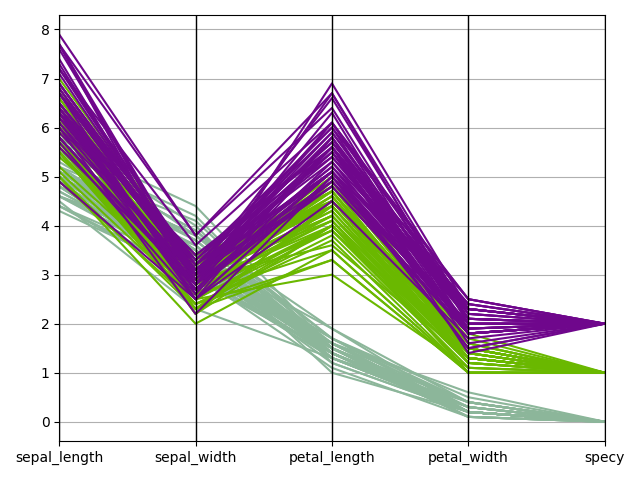# Plot - Parallel coordinates¶

from __future__ import division, unicode_literals

from matplotlib import pyplot as plt

configure_logger()


Out:

<RootLogger root (INFO)>


iris = load_dataset("IrisDataset")


### Plot parallel coordinates¶

We can use the ParallelCoordinates plot, a.k.a. cowebplot, where each samples is represented by a continuous straight line in pieces whose nodes are indexed by the variables names and measure the variables values.

iris.plot("ParallelCoordinates", classifier="specy", show=False)
# Workaround for HTML rendering, instead of show=True
plt.show()Total running time of the script: ( 0 minutes 0.385 seconds)

Gallery generated by Sphinx-Gallery